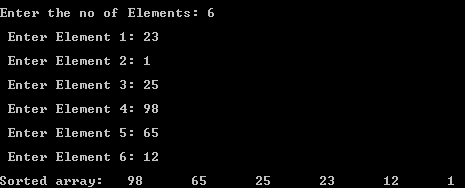## Thursday, 8 January 2015

### C Program to arrange the elements in an array in Descending Order

This is a C Program which arranges all the elements in an array in descending order.

Here initially first element in array and  its next element are compared array and array , if array is less, then the values are swapped, and the process continues with 2 and 3 elements and son on until the end of array is reached.

Finally the obtained array is the sorted array in descending order.

PROGRAM :
```#include<stdio.h>
#include<conio.h>
int main()
{
int i,j,no,temp;

printf("\nEnter the no of Elements: ");
scanf("%d", &no);
int arr[no];
for(i=0;i<no;i++)
{
printf("\n Enter Element %d: ", i+1);
scanf("%d",&arr[i]);
}

for(i=0;i<no;i++)
{
for(j=i;j<no;j++)
{
if(arr[i] < arr[j])
{
temp=arr[i];
arr[i]=arr[j];
arr[j]=temp;
}
}
}

printf("\nSorted array:");
for(i=0;i<no;i++)
printf("\t%d",arr[i]);

return 0;
}```

OUTPUT :C - Descending Order

Related Programs :